# Critical angle refractometer

How does the critical angle refractometer work? There are several types and approaches to making a device that uses critical angle, so there is no one answer to the question. Lets take a look at the most popular model, Abbé refractometer.

First, let's look at how the light refracts in a prism. We put a light source below the prism and observe what is happening. Depending on the exact light source position, light enters the prism at different angles: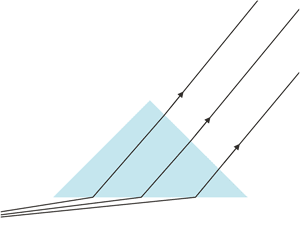Refraction in the prism - light source far to the left.

When the source of light is far to the left, ligh beam approaches the surface at a grazing angle. And this is the most important part of the story. As you know, at critical angle refracted light ray ends traveling parallel to the surface. That also means that ray that is parallel to the surface - if it happens to enter the prism - will be refracted at exactly critical angle. There are no light rays that will enter the prism at larger angle, and all other refracted rays will be more perpendicular to the prism surface.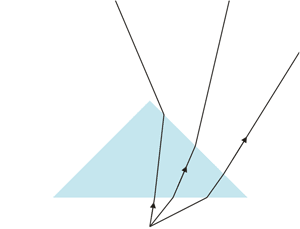Refraction in the prism - light source below prism.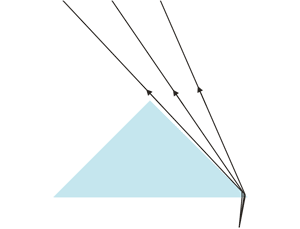Refraction in the prism - light source on the right.

Note, that if we move the light source further right, ligh entering the prism will be refracted in such a way that it will not reach the right upper surface, so even if it will leave the prism, we will be not able to observe it from this side.

What will happen if we combine these refracted rays on one picture?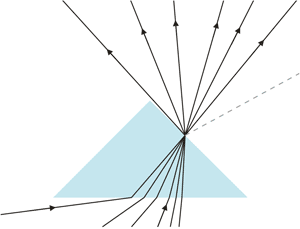Refraction in the prism - combining various light sources.

As you see, there is plenty of rays refracted and exiting the prism, but they all are on one side of the ray that is defined by the critical angle. There are no rays entering the prism from below, that can get refracted and leave the prism in the direction shown by dotted gray line. Each point of the prism surface is similar source of light - all exiting rays go "up", none goes "down". This looks more or less like this: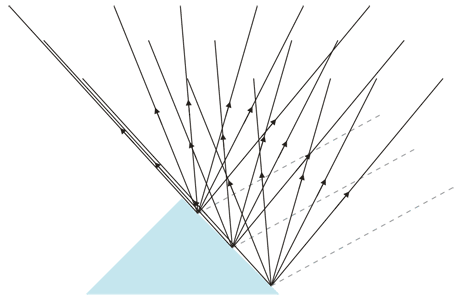Refraction in the prism - ligh exiting upper prism surface.

That explains what we see when we look at prism at the proper angle: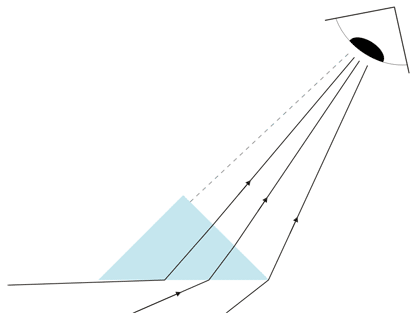Refraction in the prism - what can be seen.

There is plenty of refracted rays leaving lower part of the prism surface and travelling in the direction of the eye, so we can easily see through the prism. Depending on what is below that can mean just a bright surface, not necesarilly printed copy of the Snell's law web page. But there is no light travelling in the direction of the observer from the upper part of the prism surface, so this part looks dark. That's why the prism looks like on this picture: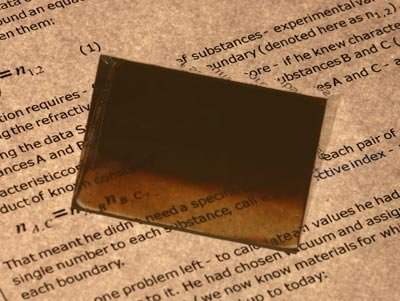Critical angle observed through a prism as a border between bright and dark area.

Angle at which the border is visible depends on the critical angle at the bottom surface of the prism. Images above are all drawn assuming prism is just in the air, also the picture was taken in the air, but if the prism rests on some liquid, critical angle will be different, and all rays shown will take slightly different paths. However, observed effect will be identical, just the angle at which we will see the border between light and dark will be different. Knowing the angle at which the border is visible, we can relatively easily calculate critical angle and refractive index of the liquid wetting bottom surface of the prism. And - after all - finding refractive index is what the crtitical angle refractometer is designed for.

That was working principle of Abbé refractometer, what about Pulfrich refractometer?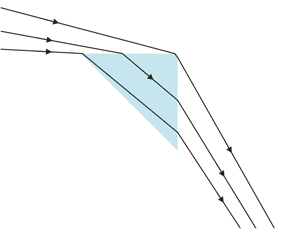Refraction in the prism - Pulfrich refractometer.

Position of the prism and measured liquid is different, but the general idea is very similar. Light is shined at the very small grazing angle, and it enters prism at critical angle. It gets refracted once again at the other prism surface, and - for exactly the same reasons as you have seen above - when looking at correct angle you will see the border between light and dark areas. Angle at which the border is visible depends on the critical angle at the upper prism surfacem, which allows easy calculation of its value.